# High School Physics : Understanding Wavelength and Frequency

## Example Questions

1 3 Next →

### Example Question #21 : Understanding Wavelength And Frequency

A saxophone plays a B-flat at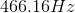in normal air at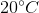. What is the wavelength of the note?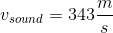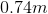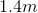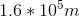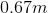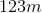Explanation:

Remember that the velocity of a wave is always equal to the wavelength times the frequency: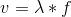All sound waves will travel with the same velocity, the speed of sound. Using this value and the given frequency, we can calculate the wavelength.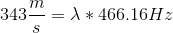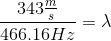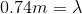### Example Question #22 : Understanding Wavelength And Frequency

A flute plays a note with a frequency of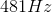. What is the period of this note?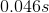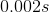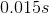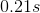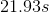Explanation:

Period is the inverse of frequency: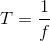.

Given the frequency, we simply need to take the reciprocal in order to find the period.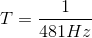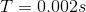1 3 Next →